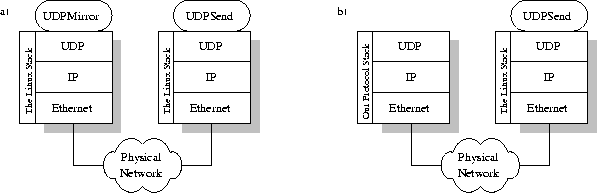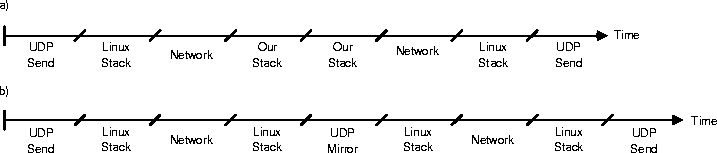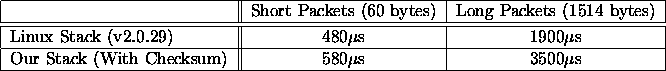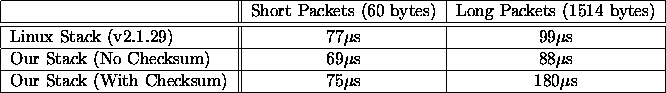Next: 5 Conclusions Up: Implementing a High Performance Previous: 3 A TCP/IP Stack

# 4 Performance Evaluation

The performance of a protocol stack is affected by its implementation. Small changes to the implementation might affect the performance considerably. An example of this is CRA. Furthermore, the performance is hard to measure, and to compare two protocol stacks are even harder. The following sections describe the methods we devised to measure the stack performance.

## 4.1 Description

To achieve something useful from the measurements, one stack has to be compared to another, preferably many others. The problem is that the time spent in the stack is hard to isolate from the total time it takes to transfer a packet. One way to solve this problem is to use a setup according to the one in figure 14. With this setup the only thing that changes when measuring a new stack, is the stack itself (and the use of the UDPMirror program for the new stack, but this does not take much time).Figure 14: a) Using Linux as mirroring stack. b) Using our stack as mirroring stack.

To help us complete this setup we wrote two programs, intended to be run on top of a normal Unix protocol stack. The first of them was UDPmirror. UDPmirror takes an incoming UDP packet and returns it to the sender. UDPmirror is implemented on the layer above UDP, using UDP to send the packets back. The other program we used was UDPSend, which is a program that sends a specific amount of UDP packets to another host. It sends one packet, and measures the time until it is returned, then it sends another packet and so on.

The complete specification of how we setup our test system can be found in appendix B.

### The Round-Trip Time

The first performance test we made was to measure the round-trip time for a packet. This was the time it took the packet to travel from the sending host, down through its protocol stack, over the network, up through the protocol stack of the receiving host, and then back again. We used the UDPSend program to send 10000 short packets (consisting of 60 bytes, the minimum with Ethernet) and measure the time it took for them to return. Then we sent 1000 long packets (consisting of 1514 bytes, the maximum with Ethernet) and measured the same time. We performed these measurements a number of times to come up with the mean values below.

First we used the setup in figure 14a to measure the round-trip time with the Linux stack as mirroring stack. Then we used the setup in figure 14b to do the same measurement, but this time using our stack as the mirroring one.

Dividing these times by the number of sent packets gives us an approximate time for the round-trip time of one packet. In the first measurement this is the time to pass the Linux stack four times, and the network twice plus the time in the UDPMirror and UDPSend programs (see figure 15a). In the second measurement it is the time to pass through the Linux stack and our stack twice, and to pass the network two times plus the time in the UDPSend program (see figure 15b). Included in the time for the Linux stack is two context switches between the stack and the UDPMirror program, and included in the time for our stack is two context switches between the kernel and our stack, which evens out this extra time. So the difference in time between the two measurements should be how much longer (or shorter) it takes to pass up and down though our stack minus the time in the UDPMirror program. The time in the UDPMirror program, however, is negligible.Figure 15: a) Times using Linux as mirroring stack. b) Times using our stack as mirroring stack. (These time lines are not drawn to scale.)

This measurement gives us a comparison between our stack and the stack in Linux. However, the measurement is inexact in that the loads on the machines may vary even if all unnecessary processes have been stopped, which may affect the results. Also, the load on the network may affect the results. Therefore we connected our test computers to each other, but had them disconnected from the rest of the network.

Our belief was that this simple measurement would give us a first approximation of how fast our protocol stack was, and we hoped to show that our stack was comparable in speed (or faster) than the Linux protocol stack, i.e. that the time spent executing in the stack would be as short as possible.

The results from the measurement of round-trip times are in table 16. These show that the round-trip time for long packets is almost the double for our stack compared to the Linux stack, but for short packets it is only about 20 per cent longer.Figure 16: The round-trip times for a packet with our stack and the Linux stack

### 4.1.2 The Time in the Stack

To better measure just the time spent in the protocol stack under test we devised a more accurate way of doing the measurements. We still used the same way as in the previous test to send and receive packets, but we connected an oscilloscope to the parallel port of the mirroring host. Then we modified our stack so that when it received a packet it raised one of the data bits on the parallel port. Later, when it send a packet it lowered the data bit again. By using the oscilloscope we could now measure the time the data bit was raised, giving us the time it took the packet to travel up and down through the stack.

We also had to modify the Linux kernel so that it too raised and lowered a data bit on the parallel port in the same way as our stack did. Unfortunately this method of measuring the time for the Linux stack includes the time to do the context switch between the kernel and the UDPMirror program, and back again. This might affect the result to the worse for the Linux stack.

Further, we wanted to verify the fact that removing the calculation of the UDP checksum would significantly cut down the time for the processing of a packet (as discussed in section 3.6). Therefore we also performed this measurement with a special version of our stack which did not do any checksum calculations on the UDP level.

The results from the measurement of the actual time spent in the protocol stack are in table 17. These indicate that our stack actually performs as well as the Linux stack, with the exception of long packets with the checksum enabled. The reason that Linux performs so much better for long packets is that we do checksumming and copying of the packet in two separate steps, requiring touching the data twice, while Linux does it in one combined step.Figure 17: The times spent in our stack and in the Linux stack for a packet

## 4.2 Discussion

There are a couple of aspects affecting the measurements, some advantages, some disadvantages to our stack. The major advantage for our stack is on the UDP level. The packets in the Linux stack are mirrored on the level above UDP, in our stack the packets return on the UDP level right after they have been decoded. The major disadvantage for our stack is that it runs as a user process. The Linux stack runs in the kernel, and therefore has better conditions. Another major disadvantage for our stack is that the Linux stack runs in parallel to our stack fighting for the time to process the packets. The stack in the Linux kernel does not process the packet all the way through the stack. After decoding the packet at the IP level it realizes that the IP number does not match the computer's IP number, and discards the packet. Unfortunately there is no way to turn of the Linux stack and still have the computer remain being usable.

A way to eliminate the effects of the Linux stack while measuring on our stack would have been to simulate the network. This can be done by either reading preformatted packets from a file, or just using one packet over and over again.

The second method we used to measure the performance of our stack is very similar to the one used in  which we later found out. The main difference between the two methods is that in  a logic analyzer is used to measure different times for various tasks in the stack, whereas we use an oscilloscope to measure the complete time in the stack.Next: 5 Conclusions Up: Implementing a High Performance Previous: 3 A TCP/IP Stack

Peter Kjellerstedt
Thu Jun 5 00:52:23 MET DST 1997